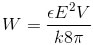﻿ Electrostatics
﻿

## Coulomb's Law

q: size of charges(c), ε: relative permittivity (in vaccum ε=1), r: distance between the charges(m)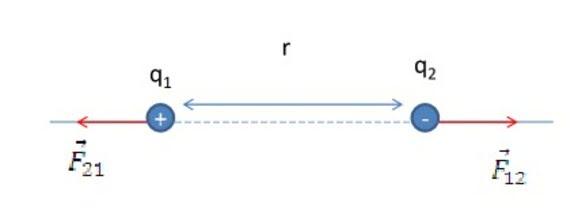## Electric Fields

E: electric field strength (N/C)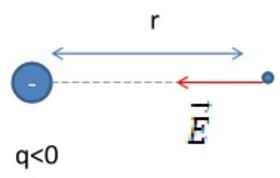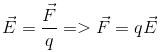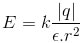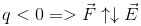## The Principle Of Superposition For electric FieldsIn case of 2 electric fields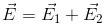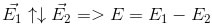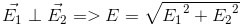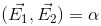## Work Of Electric Force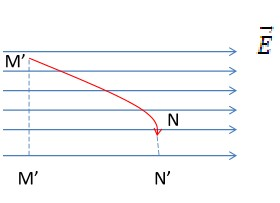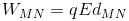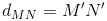## Electric Potential Difference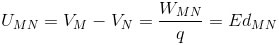## Relation Between E And U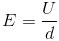## Capacitance

C: capacitance (F), Q: total electric charge(c), U: electric potential (V)## Capacitor

A: are of each electrode plate (m2), d: distance between the electrodes (m), ε: relative permittivity (F/m)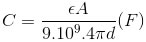## Capacitances In Series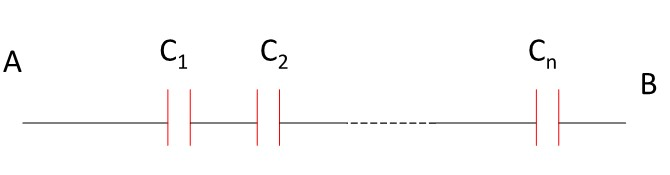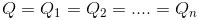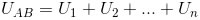## Capacitances In Parallel## Electric Field Energy## Energy Of A Capacitor

V: A.d: volume between two electrode plates